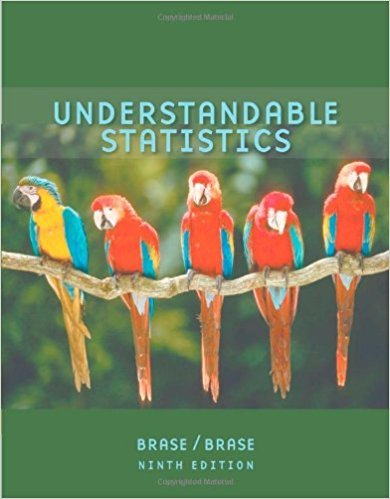×
×

# Solutions for Chapter 3.2: Averages and Variation## Full solutions for Understandable Statistics | 9th Edition

ISBN: 9780618949922Solutions for Chapter 3.2: Averages and Variation

Solutions for Chapter 3.2
4 5 0 363 Reviews
21
5
##### ISBN: 9780618949922

Chapter 3.2: Averages and Variation includes 21 full step-by-step solutions. This expansive textbook survival guide covers the following chapters and their solutions. Since 21 problems in chapter 3.2: Averages and Variation have been answered, more than 35325 students have viewed full step-by-step solutions from this chapter. This textbook survival guide was created for the textbook: Understandable Statistics, edition: 9. Understandable Statistics was written by and is associated to the ISBN: 9780618949922.

Key Statistics Terms and definitions covered in this textbook
• 2 k p - factorial experiment

A fractional factorial experiment with k factors tested in a 2 ? p fraction with all factors tested at only two levels (settings) each

• Alias

In a fractional factorial experiment when certain factor effects cannot be estimated uniquely, they are said to be aliased.

• Alternative hypothesis

In statistical hypothesis testing, this is a hypothesis other than the one that is being tested. The alternative hypothesis contains feasible conditions, whereas the null hypothesis speciies conditions that are under test

• Contingency table.

A tabular arrangement expressing the assignment of members of a data set according to two or more categories or classiication criteria

• Continuous random variable.

A random variable with an interval (either inite or ininite) of real numbers for its range.

• Crossed factors

Another name for factors that are arranged in a factorial experiment.

• Cumulative distribution function

For a random variable X, the function of X deined as PX x ( ) ? that is used to specify the probability distribution.

• Defect

Used in statistical quality control, a defect is a particular type of nonconformance to speciications or requirements. Sometimes defects are classiied into types, such as appearance defects and functional defects.

• Deming’s 14 points.

A management philosophy promoted by W. Edwards Deming that emphasizes the importance of change and quality

• Density function

Another name for a probability density function

• Dependent variable

The response variable in regression or a designed experiment.

• Designed experiment

An experiment in which the tests are planned in advance and the plans usually incorporate statistical models. See Experiment

• Discrete random variable

A random variable with a inite (or countably ininite) range.

• Estimate (or point estimate)

The numerical value of a point estimator.

• Expected value

The expected value of a random variable X is its long-term average or mean value. In the continuous case, the expected value of X is E X xf x dx ( ) = ?? ( ) ? ? where f ( ) x is the density function of the random variable X.

• Exponential random variable

A series of tests in which changes are made to the system under study

• Extra sum of squares method

A method used in regression analysis to conduct a hypothesis test for the additional contribution of one or more variables to a model.

• Fixed factor (or fixed effect).

In analysis of variance, a factor or effect is considered ixed if all the levels of interest for that factor are included in the experiment. Conclusions are then valid about this set of levels only, although when the factor is quantitative, it is customary to it a model to the data for interpolating between these levels.

• Forward selection

A method of variable selection in regression, where variables are inserted one at a time into the model until no other variables that contribute signiicantly to the model can be found.

• Fraction defective control chart

See P chart

×